## Thursday, 7 August 2014

### chapter-22 Introduction to Probability Distributions

One of the most important probability distributions at this level is the Binomial distribution, which is the subject of this article.
Binomial distributions occur in relation to those experiments that are binary in nature, i.e., whose outcomes can be grouped into two classes, say, success and failure, or, say,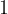$1$ and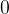$0$. For example, when you toss a coin, there are only two outcomes possible: Heads (which you may call success) and Tails (which then becomes Failure). Note that an experiment need not have only two outcomes for it to be called binary. For example, if you consider the experiment of rolling a die and make the following definitions.
 Success : Numbers$1$,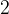$2$, or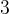$3$ Failure : Numbers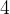$4$,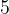$5$ and$6$
then, with respect to this definition, the experiment is binary. Thus, an experiment needs to have two classes of outcomes for it to be called binary. From now onwards, we consider the general experiment with two outcomes: success and failure, such that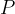$P$(Success)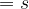$= s$$P$(Failure)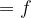$= f$
Every time such an experiment is repeated, we say that a trial of the experiment has been performed. Note that the outcome of any trial is independent of the outcome of any other trial, because the probabilities of success and failure for each trial are fixed. Such trials are also given the name Bernoulli trials.
Let us then formally state the conditions that Bernoulli trials should satisfy:
 (1) There should be a finite number of trials (2) The trials should be mutually independent (3) Each trial should have exactly two outcomes; call them success and failure. Their probabilities for every trial should be the same.
As an example, consider the experiment of tossing a fair coin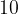$10$ times, with Heads being termed success on each toss. Thus, this experiment consists of$10$Bernoulli trials such that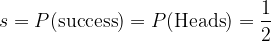$s = P({\rm{success}}) = P({\rm{Heads}}) = \dfrac{1}{2}$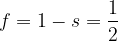$f = 1 - s = \dfrac{1}{2}$
We’ll now try to understand how the word “binomial” comes up in relation to Bernoulli trials.
Consider a sequence of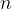$n$ Bernoulli trials with probabilities of success and failure on each trial being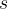$s$ and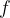$f$ respectively. We’ll now pose some question that will amply justify the word ‘Binomial’:
What is the probablility of$n$ success?
This is the probability that we obtain success on every trial, i.e.,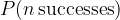$P(n \,{\rm{successes}})$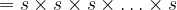$= s \times s \times s \times \ldots \times s$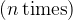$(n \,{\rm{times}})$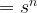$= {s^n}$
What is the probability of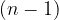$(n - 1)$ successes?
This is the probability that there should be a failure on any one trial, with the rest being successes. The failure can be on any one trial in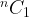${}^n{C_1}$ ways, so that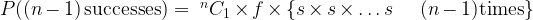$P((n - 1)\,{\rm{successes}})= {\;^n}{C_1} \times f \times \{ s \times s \times \ldots s\,\,\,\,\,\,\,\,(n - 1){\rm{times}}\}$$= {\;^n}{C_1}\;{s^{n - 1}}f$
What is the probability of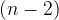$(n - 2)$ successes?
This is the probability that there should be failures on any two trials, which can happen in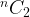${}^n{C_2}$ ways, and the rest should be successes, so that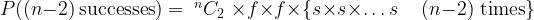$P((n - 2)\,{\rm{successes}}) = {\;^n}{C_2}\; \times f \times f \times \{ s \times s \times \ldots s\,\,\,\,\,\,\,(n - 2)\;{\rm{times}}\}$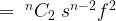$= {\;^n}{C_2}\;{s^{n - 2}}{f^2}$
Continuining in this way, we see that the probability of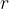$r$ successes in$n$Bernoulli trials is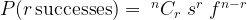$P(r \,{\rm{successes}}) = {\;^n}{C_r}\;{s^r}\;{f^{n - r}}$
which is actually the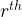${r^{th}}$ term in the Binomial expansion of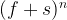${(f + s)^n}$. This is the reason for the distribution being called BinomialFor example, in case of$3$ Bernoulli trials, we have